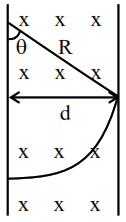# Two ions of masses 4

Question:

Two ions of masses 4 amu and 16 amu have charges $+2 \mathrm{e}$ and $+3 \mathrm{e}$ respectively. These ions pass through the region of constant perpendicular magnetic field. The kinetic energy of both ions is same. Then :

1. lighter ion will be deflected less than heavier ion

2. lighter ion will be deflected more than heavier ion

3. both ions will be deflected equally

4. no ion will be deflected.

Correct Option: , 2

Solution:

$\mathrm{r}=\frac{\mathrm{P}}{\mathrm{qB}}=\frac{\sqrt{2 \mathrm{mk}}}{\mathrm{qB}}$

Given they have same kinetic energy

$r \propto \frac{\sqrt{m}}{q}$

$\frac{r_{1}}{r_{2}}=\frac{\sqrt{4}}{2} \times \frac{3}{\sqrt{16}}=\frac{3}{4}$

$\mathrm{r}_{2}=\frac{4 \mathrm{r}_{1}}{3}$( $r_{2}$ is for hearier ion and $r_{1}$ is for lighter ion)$\sin \theta=\frac{d}{R}$

$\theta \rightarrow$ Deflection

$\theta \propto \frac{1}{\mathrm{R}}$

$(\mathrm{R} \rightarrow$ Radius of path $)$

$\because \mathrm{R}_{2}>\mathrm{R}_{1} \Rightarrow \theta_{2}<\theta_{1}$